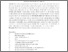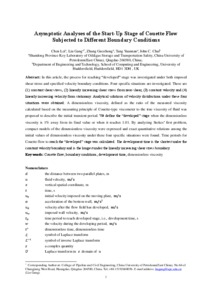Chen, Lei, Liu, Gang, Zhang, Guozhong, Tang, Yuannan and Chai, John (2017) Asymptotic Analyses of the Start-Up Stage of Couette Flow Subjected to Different Boundary Conditions. International Communications in Heat and Mass Transfer, 88. pp. 177-182. ISSN 0735-1933
Abstract

In this article, the process for reaching “developed” stage was investigated under both imposed shear stress and specified velocity boundary conditions. Four specific situations are investigated. These are (1) constant shear stress, (2) linearly increasing shear stress from zero shear, (3) constant velocity and (4) linearly increasing velocity from stationary. Analytical solutions of velocity distributions under these four situations were obtained. A dimensionless viscosity, defined as the ratio of the measured viscosity calculated based on the measuring principle of Couette-type viscometer to the true viscosity of fluid was proposed to describe the initial transient period. We define the “developed” stage when the dimensionless viscosity is 1% away from its final value or when it reaches 1.01. By analyzing Stokes' first problem, compact models of the dimensionless viscosity were expressed and exact quantitative relations among the initial values of dimensionless viscosity under these four specific situations were found. Time periods for Couette flow to reach the “developed” stage was calculated. The development time is the shortest under the constant velocity boundary and is the longest under the linearly increasing shear stress boundary.

Information
Library
DocumentsPreview
Accepted Manuscript.pdf - Accepted Version